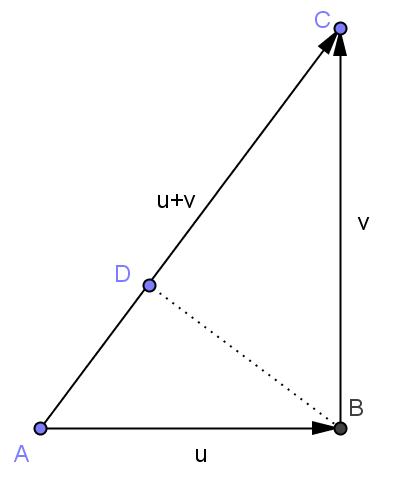# 用向量的内积证明勾股定理——体会代数的威力

||u+v||²=<u+v,u+v>=<u,u+v>+<v,u+v>=<u,u>+<u,v>+<v,u>+<v,v>。4月27日有关余弦定理的补充：

||u-v||²=<u-v,u-v>=<u,u>+<v,v>-<u,v>-<v,u>=||u||²+||v||²-2<u,v>
||u+v||²=<u+v,u+v>=<u,u>+<v,v>+<u,v>+<v,u>=||u||²+||v||²+2<u,v>

<u,v>=<v,u>=0，和勾股定理||u-v||²=||u+v||²=||u||²+||v||²

# 向量外积与行列式的本质关系

1) |a×b|=|a||b|sin<a,b> 其中sin<a,b>表示a和b两向量夹角的正弦。
2) 若|a×b|≠0，那么a×b的方向垂直于a和b所在平面并且使a,b,c满足右手螺旋定则。

1) a×b = -b×a
2) (a+b)×c = a×c+b×c
3) a×(b+c) = a×b+a×c
4) a×(kb)=(ka)×b=k(a×b)
5) i×j=k,j×k=i,k×i=j

$$\displaystyle \textbf{a}\times \textbf{b}=(a_1i+a_2j+a_3k)\times (b_1i+b_2j+b_3k)$$

$$\displaystyle \textbf{c}=( \begin{vmatrix}a_2&a_3\\b_2&b_3\end{vmatrix},-\begin{vmatrix}a_1&a_3\\b_1&b_3\end{vmatrix},\begin{vmatrix}a_1&a_2\\b_1&b_2\end{vmatrix})$$

\begin{aligned}\textbf{c}\cdot\textbf{a}&=a_1\begin{vmatrix}a_2&a_3\\ b_2&b_3\end{vmatrix}-a_2\begin{vmatrix}a_1&a_3\\ b_1&b_3\end{vmatrix}+a_3\begin{vmatrix}a_1&a_2\\ b_1&b_2\end{vmatrix}\\ &=\begin{vmatrix} a_1&a_2&a_3\\ a_1&a_2 &a_3\\ b_1&b_2&b_3\end{vmatrix}\\ &=0\end{aligned}

\begin{aligned}\textbf{c}\cdot\textbf{b}&=b_1\begin{vmatrix}a_2&a_3\\ b_2&b_3\end{vmatrix}-b_2\begin{vmatrix}a_1&a_3\\ b_1&b_3\end{vmatrix}+b_3\begin{vmatrix}a_1&a_2\\ b_1&b_2\end{vmatrix}\\ &=\begin{vmatrix} b_1&b_2&b_3\\ a_1&a_2 &a_3\\ b_1&b_2&b_3\end{vmatrix}\\ &=0\end{aligned}

| a1 a2 a3 |
| b1 b2 b3 |
| c1 c2 c3 |

| a1 a2 a3 |
| b1 b2 b3 |
| c1 c2 c3 |

|c| ² =|a| |b| sin<a,b> |c| (因为体积等于底面积乘以高)

| a1 a2 a3 |
| b1 b2 b3 |
| d1 d2 d3 |

a1,a2, …,an-1,a1×a2×…an-1取正向，那么必须在表示外积的行列式中把基底那一行写在最下面，然后按照基底那一行展开成各个分量。

1）行列式，2）3个向量张成多面体的有向体积，3）2个向量的外积，4）3个向量的混合积